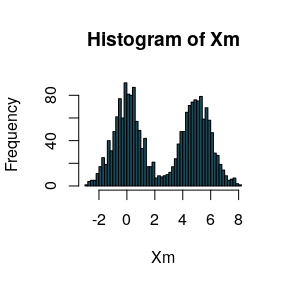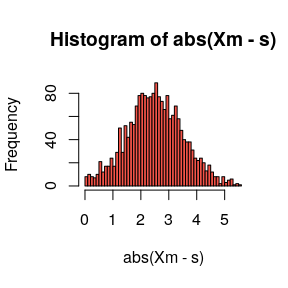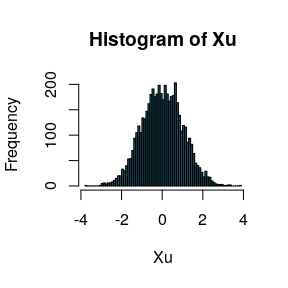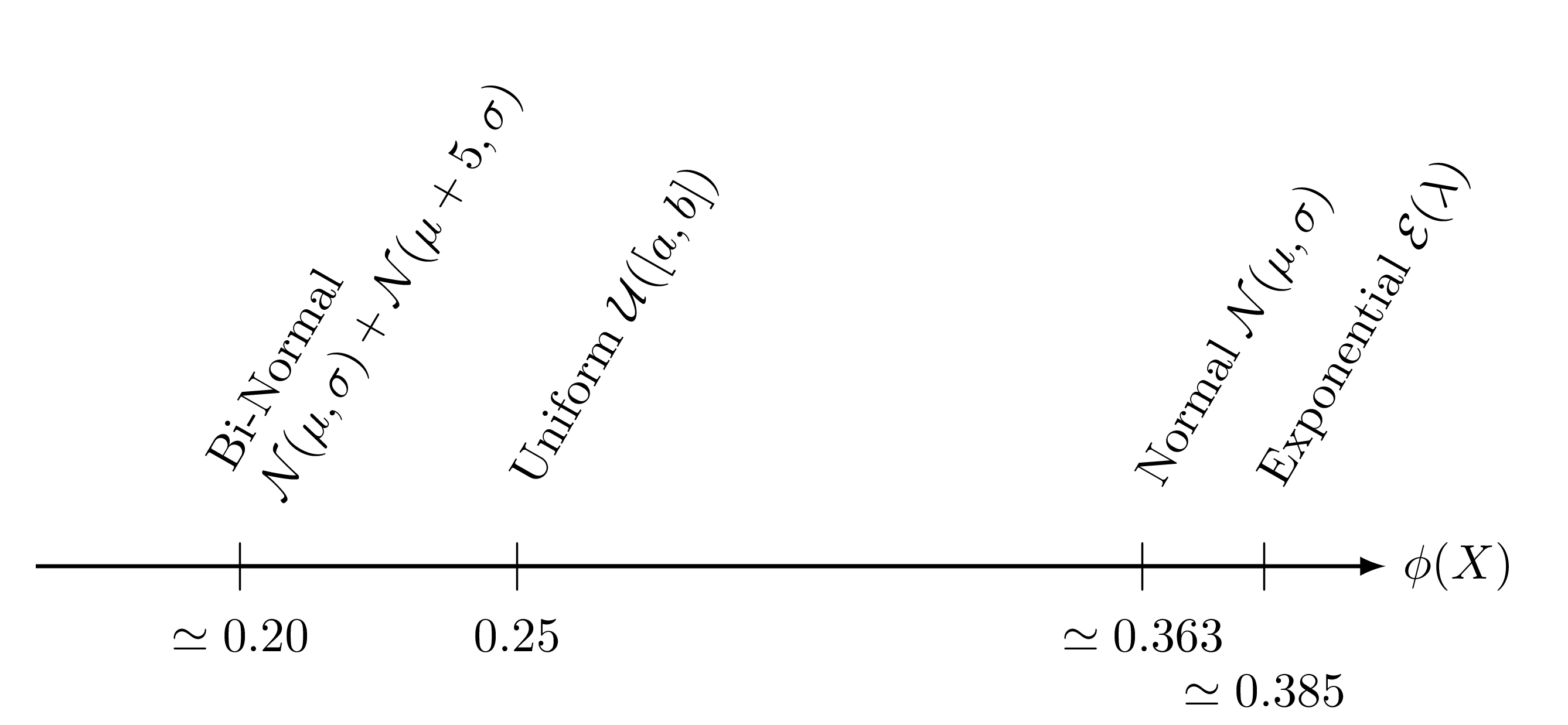Internals

2018-09-19

In this vignette, we describe the founding principles of the folding test of unimodality. Both the initial intuition and the mathematical formulation are presented (with some examples).

Intuition

Let us have a look on a bimodal distribution (two normal modes)

Xm = c(rnorm(1000,0,1),rnorm(1000,5,1))
hist(Xm, freq=TRUE, nclass=60, col="#1B4B5A")Because of the two modes, data are far from the mean, so the variance of the distribution is quite large.

print(var(Xm))
##  7.362888

The idea is the following: if we can fold the first mode into the second, the final variance will be reduced. To fold correctly, we have to find the best folding axis $$s^*$$ (called folding pivot). In our case, it should be close to 2.5.

If we fold the density along $$s^*$$, the resulting density (the density of $$|X-s^*|$$) looks like this:

s = 2.5
hist(abs(Xm - s), freq=TRUE, nclass=60, col="#F55449")Now, if we compute its variance we get 1.0112503, which is far lower. In fact this phenomenon will not appear when the initial distribution is unimodal. Let us take a simple standard normal law:

Xu = rnorm(5000,0,1)
hist(Xu, freq=TRUE, nclass=60, col="#1B4B5A")We know that its variance is close to 1. However, the folding point is not as clear as in the bimodal case. That’s why we are likely to find the best one (those which reduce the variance the most): $s^* = \underset{s\in\mathbb{R}}{\operatorname{argmin}} \operatorname{Var}\left|X-s\right|$

f = function(s) {var(abs(Xu-s))}
optim_results = optim(2.0, f, method="BFGS")
s_star = optim_results\$par
print(s_star)
##  0.01803807

Actually, the best folding pivot is likely to be close to the mode (in the symmetrical case, the mode is the best folding pivot). Now if we look at the folded variance (the variance of the folded distribution), we get 0.3519293. Obviously, the variance is lower than the initial one but not with the same amplitude as in the bimodal case. The folding test of unimodality is based on this mechanism.

Mathematical formulation

Here, we give some technical details about the folding process.

The pivot

We have shown the expression of the folding pivot: the point which reduces the variance the most. However two problems raise:

• The existance of this argument of the minimum is not clear (especially in the multivariate case, we will tackle it below)

• Finding the pivot requires an optimization step which may be expensive and uncertain

To avoid both problems, we rather use another good candidate $s_2^* = \underset{s\in\mathbb{R}}{\operatorname{argmin}} \operatorname{Var}\left(\left(X-s\right)^2\right) = \dfrac 12 \dfrac{\operatorname{Cov}\left(X,X^2\right)}{\operatorname{Var}X}$ This pivot is well-defined (you only need a non-degenerated random variable) but more than that it has an analytical expression which is easy to compute (or even update incrementally). As an example, we can compare $$s^*$$ and $$s_2^*$$ in the two previous cases:

Distribution $$s^*$$ $$s_2^*$$
Bimodal (case 1) 2.5112129 2.5183226
Unimodal (case 2) 0.0180381 0.0175444

On these examples, we observe that $$s_2^*$$ is close to $$s^*$$.

Quantifying the folding mechanism

Once we have a good pivot we can fold along it and check if this mechanism cuts down the variance. To quantify it, we merely make the relation between the initial variance and the folded one (we call it the folding ratio): $\phi(X) = \dfrac{\operatorname{Var}\left|X-s_2^*\right|}{\operatorname{Var} X}$ So, if $$\phi(X)$$ is high, the folding does not reduce so much the variance, then the distribution is rather unimodal. On the contrary, if $$\phi(X)$$ is low, it means that the folding has a real impact, then the ditribution is rather multimodal.

To show that $$\phi(X)$$ preserves this kind of unimodality order, we can compute analytically the folding ratio for some common distributions.We notice two things:

• The distributions seem well-ranked
• The folding ratio does not depend on the distribution parameters

In a word, this ratio has good properties so as to discriminate multimodal distributions from unimodal ones.

The final decision

We can compute the folding ratio, but what is the decision bound? At which value should we consider a distribution rather unimodal/multimodal? Actually, the uniform case is commonly considered as the worst case of unimodality (see J. A. Hartigan and Hartigan 1985 for instance). We can understand that few modifications on this density can make it either unimodal or multimodal. To convince the wizards at maths, the set of the unimodal distributions is actually convex and the uniform distributions are on its frontier (Dharmadhikari and Joag-Dev 1988).

It leads us to introduce the folding statistics: $\Phi(X) = \dfrac{\phi(X)}{\phi(U)} = 4~\phi(X)$ where $$U$$ is a uniform random variable (we have seen that the parameters do not matter at all). Finally we present the Folding Test of Unimodality:

• If $$\Phi(X)\ge 1$$, then it means that $$\phi(X)\ge \phi(U)$$, so $$X$$ is rather unimodal
• If $$\Phi(X)< 1$$, then it means that $$\phi(X)< \phi(U)$$, so $$X$$ is rather multimodal

Higher dimensions

Previously, we only tackle the univariate case but it may be easily extended to the multivariate case. Below is a table showing the formulas.

Univariate Multivariate
$\phi(X) = \dfrac{\operatorname{Var}|X-s_2^*|}{\operatorname{E}\left|X-\operatorname{E}(X)\right|^2}$ $\phi(X) = \dfrac{\operatorname{Var} \|X-s_2^*\|}{\operatorname{E}\left\|X-\operatorname{E}(X)\right\|^2}$
$s_2^* = \dfrac 12 \dfrac{\operatorname{Cov}\left(X,X^2\right)}{\operatorname{Var} X}$ $s_2^* = \dfrac 12 \Sigma^{-1}\operatorname{Cov}\left(X,\|X\|^2\right)$

The concept are identical. However, we have to precise that the folding ratio for the uniform density in dimension $$d$$ is given by $\phi(U) = \dfrac{1}{(1+d)^2}$ So the general expression of the folding statistics is the following: $\Phi(X) = (1+d)^2 \phi(X)$